Go through the Math in Focus Grade 8 Workbook Answer Key Chapter 1 Exponents to finish your assignments.

## Math in Focus Grade 8 Course 3 A Chapter 1 Answer Key Exponents

### Math in Focus Grade 8 Chapter 1 Quick Check Answer Key

Identify the integers in the list below.

Question 1.
4.31, -6, 8.12, 58, -211,43
-211,-6,43 and 58,

Explanation:
Integers are positive,negative and zero but not decimal numbers therefore
from the given numbers 4.31 and 8.12 are not integers
the integers are -211,-6,43 and 58.

Write each rational number as a terminating decimal.

Question 2.
$$\frac{3}{5}$$
0.6,

Explanation: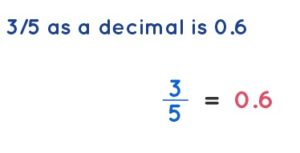when we divide 3 by 5 we get terminating decimal as 0.6.

Question 3.
4$$\frac{1}{4}$$
4.25,

Explanation:
Given 4$$\frac{1}{4}$$ we get $$\frac{4 x 4 + 1}{4}$$ =
$$\frac{17}{4}$$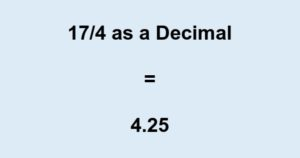the terminating decimal is 4.25.

Write each rational number as a repeating decimal.

Question 4.
$$\frac{6}{11}$$
The repeating decimal of 6/11 is 0.5363636 and so on,

Explanation:
Given to find $$\frac{6}{11}$$ we get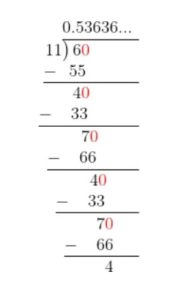the repeating decimal of 6/11 is 0.5363636 and so on.

Question 5.
$$\frac{5}{12}$$
The repeating decimal of 5/12 is 0.4166 so on,

Explanation: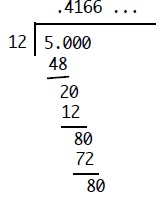The repeating decimal of 5/12 is 0.4166 so on.

Locate each irrational number on a number line using rational approximation.

Question 6.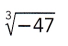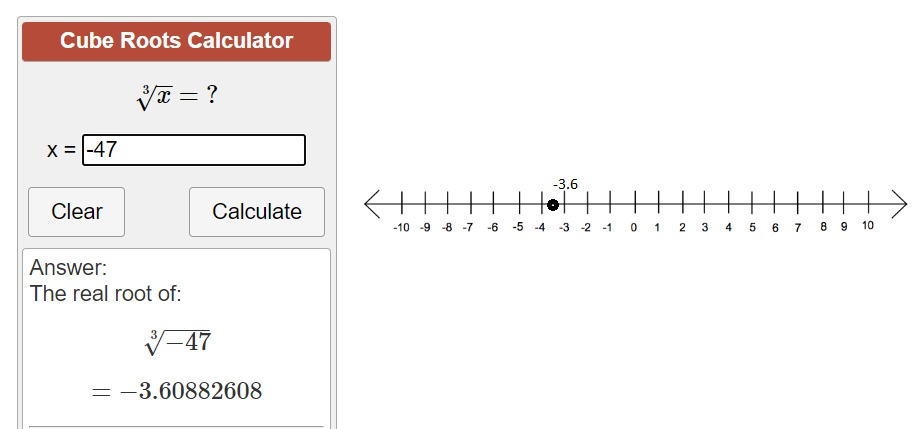Explanation:
Located irrational number cube root of -47 on the
number line – 3.6 using rational approximation.

Question 7.
$$\sqrt{19}$$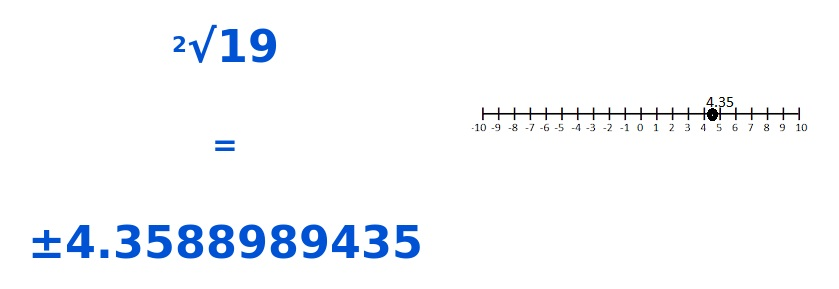Explanation:
Located irrational number square root of 19 on the
number line 4.35 using rational approximation.

Evaluate each expression.

Question 8.
-3 + (-4)
-7,

Explanation:
Adding  -3 to -4 we get -7.

Question 4.
-4 – (-2)
-6,

Explanation:
Adding -4 to -2 we get -6.

Evaluate each expression.

Question 10.
(-7) • (-3)